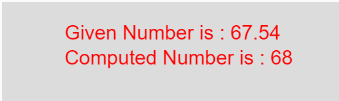# p5.js | round() function

• Last Updated : 09 Apr, 2019

The round() function in p5.js is used to calculate the round value of a number. It calculates the integer closest to the number.

Syntax

```round(number)
```

Parameters: The function accepts only one parameter as mentioned above and described below:

• number : This parameter stores the number to compute.
• Below program illustrates the round() function in p5.js:
Example:

 `function` `setup() {``    ``//create Canvas of size 270*80  ``    ``createCanvas(270, 80);``}`` ` `function` `draw() {``    ``background(220);``    ``//initialize the parameter  ``    ``let x = 67.54;``    ``//call to round() function``    ``let y = round(x);``    ``textSize(16);``    ``fill(color(``'red'``));``    ``text(``"Given Number is : "` `+ x, 50, 30);``    ``text(``"Computed Number is : "` `+ y, 50, 50);``}`

Output:Reference: https://p5js.org/reference/#/p5/round

My Personal Notes arrow_drop_up